# PostgreSQL Tutorial: Aggregate Functions

September 26, 2023

Summary: in this tutorial, you will learn how to use the PostgreSQL aggregate functions such as `AVG()`, `COUNT()`, `MIN()`, `MAX()`, and `SUM()`.

## Introduction to PostgreSQL aggregate functions

Aggregate functions perform a calculation on a set of rows and return a single row. PostgreSQL provides all standard SQL’s aggregate functions as follows:

We often use the aggregate functions with the `GROUP BY` clause in the `SELECT` statement. In these cases, the `GROUP BY` clause divides the result set into groups of rows and the aggregate functions perform a calculation on each group e.g., maximum, minimum, average, etc.

You can use aggregate functions as expressions only in the following clauses:

## PostgreSQL aggregate functions examples

Let’s use the `film` table in the sample database for the demonstration.## `AVG()` function examples

The following statement uses the `AVG()` function to calculate the average replacement cost of all films:

``````SELECT
ROUND( AVG( replacement_cost ), 2 ) avg_replacement_cost
FROM
film;
``````

The following is the result: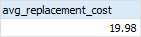Noted that the `ROUND()` function was used to round the result to 2 decimal places.

To calculate the average replacement cost of the Drama films whose category id is 7, you use the following statement:

``````SELECT
ROUND( AVG( replacement_cost ), 2 ) avg_replacement_cost
FROM
film
INNER JOIN film_category USING(film_id)
INNER JOIN category USING(category_id)
WHERE
category_id = 7;
``````

Here is the result: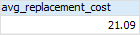## `COUNT()` function examples

To get the number of films, you use the `COUNT(*)` function as follows:

``````SELECT
COUNT(*)
FROM
film;
``````

Here is the output: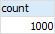To get the number of drama films, you use the following statement:

``````SELECT
COUNT(*) drama_films
FROM
film
INNER JOIN film_category USING(film_id)
INNER JOIN category USING(category_id)
WHERE
category_id = 7;
``````

The result showed that we have 62 drama films: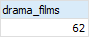## `MAX()` function examples

The following statement returns the maximum replacement cost of films.

``````SELECT
MAX(replacement_cost)
FROM
film;
``````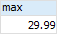To get the films that have the maximum replacement cost, you use the following query:

``````SELECT
film_id,
title
FROM
film
WHERE
replacement_cost =(
SELECT
MAX( replacement_cost )
FROM
film
)
ORDER BY
title;
``````The subquery returned the maximum replacement cost which then was used by the outer query for retrieving the film’s information.

## `MIN()` function examples

The following example uses the `MIN()` function to return the minimum replacement cost of films:

``````SELECT
MIN(replacement_cost)
FROM
film;
``````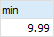To get the films which have the minimum replacement cost, you use the following query:

``````SELECT
film_id,
title
FROM
film
WHERE
replacement_cost =(
SELECT
MIN( replacement_cost )
FROM
film
)
ORDER BY
title;
``````## `SUM()` function examples

The following statement uses the `SUM()` function to calculate the total length of films grouped by film’s rating:

``````SELECT
rating,
SUM( rental_duration )
FROM
film
GROUP BY
rating
ORDER BY
rating;
``````

The following picture illustrates the result: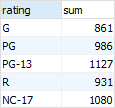In this tutorial, you have learned about PostgreSQL aggregate functions and apply them to summarize data.